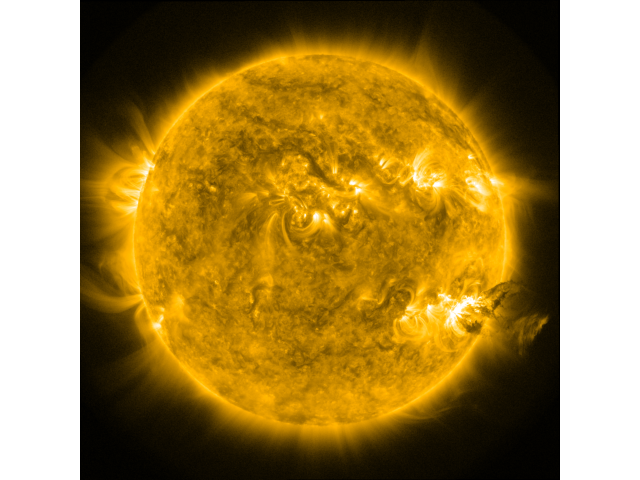# Plotting a Map without any Axes#

This examples shows you how to plot a Map without any annotations at all, i.e., to save as an image.

import matplotlib.pyplot as plt
import numpy as np

import sunpy.map
from sunpy.data.sample import AIA_171_IMAGE


Create a sunpy Map from the sample data.

smap = sunpy.map.Map(AIA_171_IMAGE)


Plot the Map without a frame. We can setup a frameless figure and an axes which spans the whole canvas.

figure = plt.figure(frameon=False)
ax = plt.axes([0, 0, 1, 1])
# Disable the axis
ax.set_axis_off()

# Plot the map.
# Since we are not interested in the exact map coordinates,
# we can simply use :meth:~matplotlib.Axes.imshow.
norm = smap.plot_settings['norm']
norm.vmin, norm.vmax = np.percentile(smap.data, [1, 99.9])
ax.imshow(smap.data,
norm=norm,
cmap=smap.plot_settings['cmap'],
origin="lower")

<matplotlib.image.AxesImage object at 0x7fb38da4e200>


At this point you could save the figure with savefig() or show it:

plt.show()Total running time of the script: (0 minutes 0.224 seconds)

Gallery generated by Sphinx-Gallery